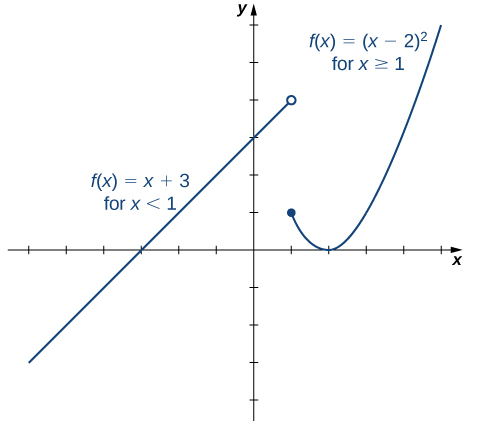# 1.2 Basic classes of functions  (Page 7/28)

 Page 7 / 28

Find the domain for each of the following functions: $f\left(x\right)=\left(5-2x\right)\text{/}\left({x}^{2}+2\right)$ and $g\left(x\right)=\sqrt{5x-1}.$

The domain of $f$ is $\text{(−∞, ∞).}$ The domain of $g$ is $\left\{x|x\ge 1\text{/}5\right\}.$

## Transcendental functions

Thus far, we have discussed algebraic functions. Some functions, however, cannot be described by basic algebraic operations. These functions are known as transcendental functions because they are said to “transcend,” or go beyond, algebra. The most common transcendental functions are trigonometric, exponential, and logarithmic functions. A trigonometric function relates the ratios of two sides of a right triangle. They are $\mathrm{sin}x,\mathrm{cos}x,\mathrm{tan}x,\mathrm{cot}x,\mathrm{sec}x,\text{and}\phantom{\rule{0.2em}{0ex}}\mathrm{csc}x.$ (We discuss trigonometric functions later in the chapter.) An exponential function is a function of the form $f\left(x\right)={b}^{x},$ where the base $b>0,b\ne 1.$ A logarithmic function    is a function of the form $f\left(x\right)={\mathrm{log}}_{b}\left(x\right)$ for some constant $b>0,b\ne 1,$ where ${\mathrm{log}}_{b}\left(x\right)=y$ if and only if ${b}^{y}=x.$ (We also discuss exponential and logarithmic functions later in the chapter.)

## Classifying algebraic and transcendental functions

Classify each of the following functions, a. through c., as algebraic or transcendental.

1. $f\left(x\right)=\frac{\sqrt{{x}^{3}+1}}{4x+2}$
2. $f\left(x\right)={2}^{{x}^{2}}$
3. $f\left(x\right)=\text{sin}\left(2x\right)$
1. Since this function involves basic algebraic operations only, it is an algebraic function.
2. This function cannot be written as a formula that involves only basic algebraic operations, so it is transcendental. (Note that algebraic functions can only have powers that are rational numbers.)
3. As in part b., this function cannot be written using a formula involving basic algebraic operations only; therefore, this function is transcendental.

Is $f\left(x\right)=x\text{/}2$ an algebraic or a transcendental function?

Algebraic

## Piecewise-defined functions

Sometimes a function is defined by different formulas on different parts of its domain. A function with this property is known as a piecewise-defined function    . The absolute value function is an example of a piecewise-defined function because the formula changes with the sign of $x\text{:}$

$f\left(x\right)=\left\{\begin{array}{c}\text{−}x,x<0\\ x,x\ge 0\end{array}.$

Other piecewise-defined functions may be represented by completely different formulas, depending on the part of the domain in which a point falls. To graph a piecewise-defined function, we graph each part of the function in its respective domain, on the same coordinate system. If the formula for a function is different for $x and $x>a,$ we need to pay special attention to what happens at $x=a$ when we graph the function. Sometimes the graph needs to include an open or closed circle to indicate the value of the function at $x=a.$ We examine this in the next example.

## Graphing a piecewise-defined function

Sketch a graph of the following piecewise-defined function:

$f\left(x\right)=\left\{\begin{array}{l}x+3,\phantom{\rule{3em}{0ex}}x<1\\ {\left(x-2\right)}^{2},\phantom{\rule{1.5em}{0ex}}x\ge 1\end{array}.$

Graph the linear function $y=x+3$ on the interval $\left(\text{−∞},1\right)$ and graph the quadratic function $y={\left(x-2\right)}^{2}$ on the interval $\left[1,\infty \right).$ Since the value of the function at $x=1$ is given by the formula $f\left(x\right)={\left(x-2\right)}^{2},$ we see that $f\left(1\right)=1.$ To indicate this on the graph, we draw a closed circle at the point $\left(1,1\right).$ The value of the function is given by $f\left(x\right)=x+2$ for all $x<1,$ but not at $x=1.$ To indicate this on the graph, we draw an open circle at $\left(1,4\right).$This piecewise-defined function is linear for x < 1 and quadratic for x ≥ 1 .

find integration of loge x
find the volume of a solid about the y-axis, x=0, x=1, y=0, y=7+x^3
how does this work
Can calculus give the answers as same as other methods give in basic classes while solving the numericals?
log tan (x/4+x/2)
Rohan
Rohan
y=(x^2 + 3x).(eipix)
Claudia
Ismael
A Function F(X)=Sinx+cosx is odd or even?
neither
David
Neither
Lovuyiso
f(x)=1/1+x^2 |=[-3,1]
apa itu?
fauzi
determine the area of the region enclosed by x²+y=1,2x-y+4=0
Hi
MP
Hi too
Vic
hello please anyone with calculus PDF should share
Which kind of pdf do you want bro?
Aftab
hi
Abdul
can I get calculus in pdf
Abdul
How to use it to slove fraction
Hello please can someone tell me the meaning of this group all about, yes I know is calculus group but yet nothing is showing up
Shodipo
You have downloaded the aplication Calculus Volume 1, tackling about lessons for (mostly) college freshmen, Calculus 1: Differential, and this group I think aims to let concerns and questions from students who want to clarify something about the subject. Well, this is what I guess so.
Jean
Im not in college but this will still help
nothing
how en where can u apply it
Migos
how can we scatch a parabola graph
Ok
Endalkachew
how can I solve differentiation?
with the help of different formulas and Rules. we use formulas according to given condition or according to questions
CALCULUS
For example any questions...
CALCULUS
v=(x,y) وu=(x,y ) ∂u/∂x* ∂x/∂u +∂v/∂x*∂x/∂v=1
log tan (x/4+x/2)
Rohan
what is the procedures in solving number 1?
review of funtion role?
for the function f(x)={x^2-7x+104 x<=7 7x+55 x>7' does limx7 f(x) exist?
no
Haliru
is derivatives of any constant no is zero
Haliru
find dy÷dx (y^2+2 sec)^2=4(x+1)^2
Integral of e^x/(1+e^2x)tan^-1 (e^x)By OpenStaxBy Robert MurphyBy OpenStaxBy Jessica CollettBy Monty HartfieldBy RhodesBy Bonnie HurstBy OpenStaxBy Michael PittBy OpenStax